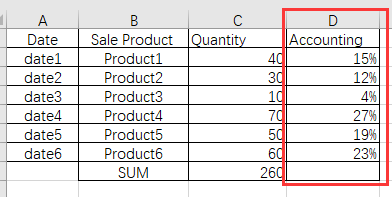# How to calculate the proportion of the number

HI，hello！
I want to calculate the ratio of the sales of a product to the total sales in a certain time range，Please tell me method，thank you！1 Like

Hi,

The easiest way for calculating percentages is to switch the report to the Pie chart giving the percentages automatically.

Other solutions might depend on how you have defined the time range for the report. The most often used calculation for percentages is by using the tuple with the Default Member of the dimension and it should work fine if you wish to see the percentages by all the periods. So, the formula for the Accounting measure could look like this:

``````[Measures].[Quantity]/
([Measures].[Quantity],
[Time].CurrentHierarchy.DefaultMember)
``````

Please, see the eazyBI documentation for more details on how to use the tuples: https://docs.eazybi.com/display/EAZYBI/Calculated+Members#CalculatedMembers-Tuples

Kindly,

1 Like